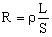# Concept of partial resistance

## Introduction

The partial resistance is defined as the resistance encountered by a uniform current which flows in a part of conductor (or partial element in the PEEC meaning).

## Definition

The formula for the computation of the partial resistance of a PEEC element comes from the Ohm's law under the hypothesis of uniform current density. Thus, it corresponds to the relationship expressing the DC-resistance of an elementary bar.

The partial resistance for a rectilinear conductor of variously shaped cross-section is then given by the following formula:where:

• ρ is the resistivity of the conductor material
• L is the length of the conductor
• S is the conductor cross-section